Skip to content
Related Articles
Python Seaborn Tutorial
• Last Updated : 10 Feb, 2021

Seaborn is a library mostly used for statistical plotting in Python. It is built on top of Matplotlib and provides beautiful default styles and color palettes to make statistical plots more attractive.In this tutorial, we will learn about Python Seaborn from basics to advance using a huge dataset of seaborn basics, concepts, and different graphs that can be plotted.

Table Of Content

Recent articles on Seaborn !!

## Getting Started

First of all, let us install Seaborn. Seaborn can be installed using the pip. Type the below command in the terminal.

`pip install seaborn`

In the terminal, it will look like this –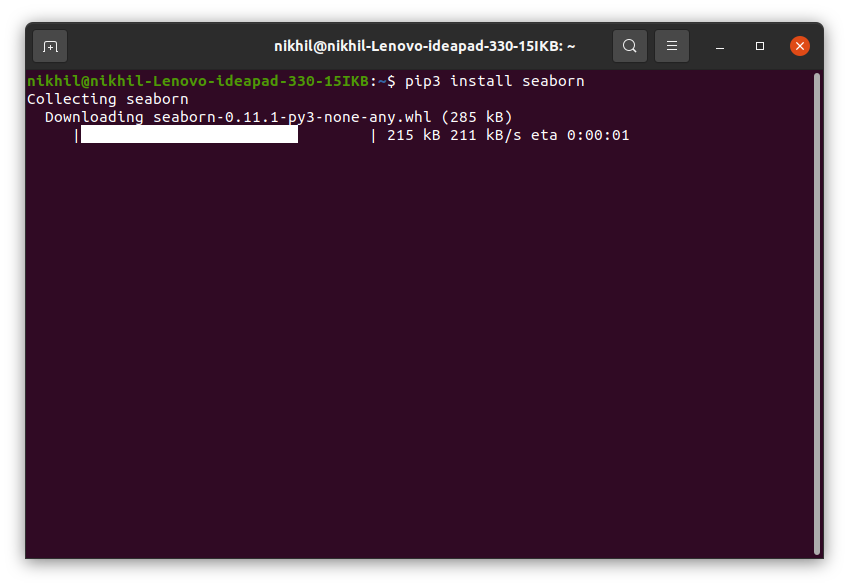After the installation is completed you will get a successfully installed message at the end of the terminal as shown below.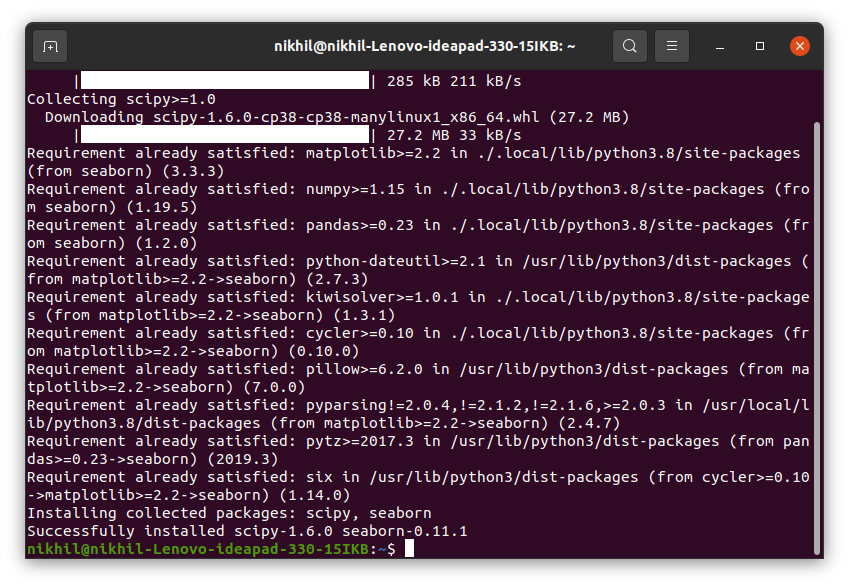Note: Seaborn has the following dependencies –

• Python 2.7 or 3.4+
• numpy
• scipy
• pandas
• matplotlib

After the installation let us see an example of a simple plot using Seaborn. We will be plotting a simple line plot using the iris dataset. Iris dataset contains five columns such as Petal Length, Petal Width, Sepal Length, Sepal Width and Species Type. Iris is a flowering plant, the researchers have measured various features of the different iris flowers and recorded them digitally.

Example:

## Python3

 `# importing packages ``import` `seaborn as sns `` ` `# loading dataset ``data ``=` `sns.load_dataset(``"iris"``) `` ` `# draw lineplot ``sns.lineplot(x``=``"sepal_length"``, y``=``"sepal_width"``, data``=``data)`

Output: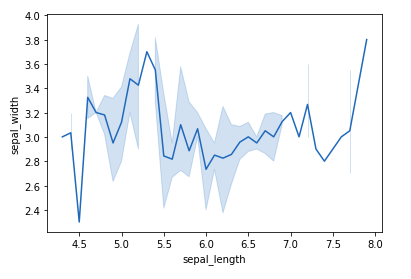In the above example, a simple line plot is created using the lineplot() method. Do not worry about these functions as we will be discussing them in detail in the below sections. Now after going through a simple example let us see a brief introduction about the Seaborn. Refer to the below articles to get detailed information about the same.

In the introduction, you must have read that Seaborn is built on the top of Matplotlib. It means that Seaborn can be used with Matplotlib.

## Using Seaborn with Matplotlib

Using both Matplotlib and Seaborn together is a very simple process. We just have to invoke the Seaborn Plotting function as normal, and then we can use Matplotlib’s customization function.

Example 1: We will be using the above example and will add the title to the plot using the Matplotlib.

## Python3

 `# importing packages ``import` `seaborn as sns ``import` `matplotlib.pyplot as plt `` ` `# loading dataset ``data ``=` `sns.load_dataset(``"iris"``) `` ` `# draw lineplot ``sns.lineplot(x``=``"sepal_length"``, y``=``"sepal_width"``, data``=``data) `` ` `# setting the title using Matplotlib``plt.title(``'Title using Matplotlib Function'``)`` ` `plt.show()`

Output: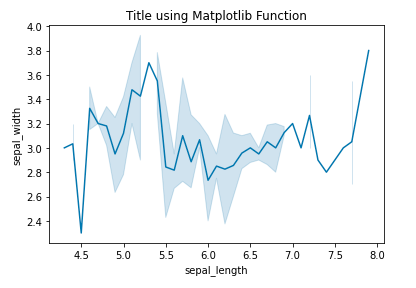Example 2: Setting the xlim and ylim

## Python3

 `# importing packages ``import` `seaborn as sns ``import` `matplotlib.pyplot as plt `` ` `# loading dataset ``data ``=` `sns.load_dataset(``"iris"``) `` ` `# draw lineplot ``sns.lineplot(x``=``"sepal_length"``, y``=``"sepal_width"``, data``=``data) `` ` `# setting the x limit of the plot``plt.xlim(``5``)`` ` `plt.show()`

Output: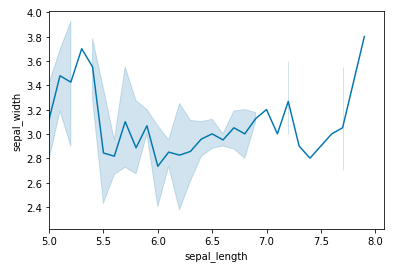## Customizing Seaborn Plots

Seaborn comes with some customized themes and a high-level interface for customizing the looks of the graphs. Consider the above example where the default of the Seaborn is used. It still looks nice and pretty but we can customize the graph according to our own needs. So let’s see the styling of plots in detail.

### Changing Figure Aesthetic

set_style() method is used to set the aesthetic of the plot. It means it affects things like the color of the axes, whether the grid is active or not, or other aesthetic elements. There are five themes available in Seaborn.

• darkgrid
• whitegrid
• dark
• white
• ticks

Syntax:

set_style(style=None, rc=None)

Example: Using the dark theme

## Python3

 `# importing packages ``import` `seaborn as sns ``import` `matplotlib.pyplot as plt `` ` `# loading dataset ``data ``=` `sns.load_dataset(``"iris"``) `` ` `# draw lineplot ``sns.lineplot(x``=``"sepal_length"``, y``=``"sepal_width"``, data``=``data) `` ` `# changing the theme to dark``sns.set_style(``"dark"``)``plt.show()`

Output: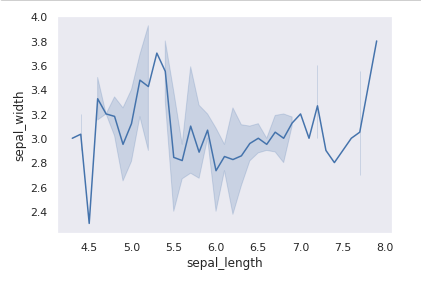### Removal of Spines

Spines are the lines noting the data boundaries and connecting the axis tick marks. It can be removed using the despine() method.

Syntax:

sns.despine(left = True)

Example:

## Python3

 `# importing packages ``import` `seaborn as sns ``import` `matplotlib.pyplot as plt `` ` `# loading dataset ``data ``=` `sns.load_dataset(``"iris"``) `` ` `# draw lineplot ``sns.lineplot(x``=``"sepal_length"``, y``=``"sepal_width"``, data``=``data) `` ` `# Removing the spines``sns.despine()``plt.show()`

Output: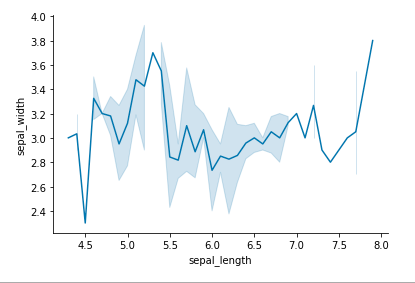### Changing the figure Size

The figure size can be changed using the figure() method of Matplotlib. figure() method creates a new figure of the specified size passed in the figsize parameter.

Example:

## Python3

 `# importing packages ``import` `seaborn as sns ``import` `matplotlib.pyplot as plt `` ` `# loading dataset ``data ``=` `sns.load_dataset(``"iris"``) `` ` `# changing the figure size``plt.figure(figsize ``=` `(``2``, ``4``))`` ` `# draw lineplot ``sns.lineplot(x``=``"sepal_length"``, y``=``"sepal_width"``, data``=``data) `` ` `# Removing the spines``sns.despine()`` ` `plt.show()`

Output: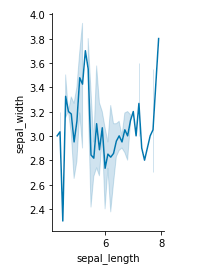### Scaling the plots

It can be done using the set_context() method. It allows us to override default parameters. This affects things like the size of the labels, lines, and other elements of the plot, but not the overall style. The base context is “notebook”, and the other contexts are “paper”, “talk”, and “poster”. font_scale sets the font size.

Syntax:

set_context(context=None, font_scale=1, rc=None)

Example:

## Python3

 `# importing packages ``import` `seaborn as sns ``import` `matplotlib.pyplot as plt `` ` `# loading dataset ``data ``=` `sns.load_dataset(``"iris"``) `` ` `# draw lineplot ``sns.lineplot(x``=``"sepal_length"``, y``=``"sepal_width"``, data``=``data) `` ` `# Setting the scale of the plot``sns.set_context(``"paper"``)`` ` `plt.show()`

Output: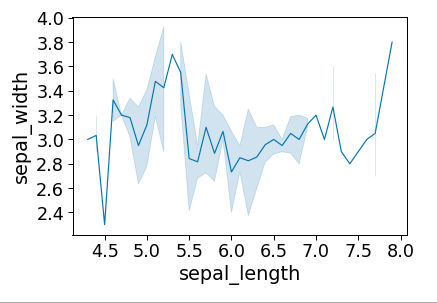### Setting the Style Temporarily

axes_style() method is used to set the style temporarily. It is used along with the with statement.

Syntax:

axes_style(style=None, rc=None)

Example:

## Python3

 `# importing packages ``import` `seaborn as sns ``import` `matplotlib.pyplot as plt `` ` `# loading dataset ``data ``=` `sns.load_dataset(``"iris"``) `` ` ` ` `def` `plot():``    ``sns.lineplot(x``=``"sepal_length"``, y``=``"sepal_width"``, data``=``data) `` ` `with sns.axes_style(``'darkgrid'``):``     ` `    ``# Adding the subplot``    ``plt.subplot(``211``)``    ``plot()``     ` `plt.subplot(``212``)``plot()`

Output: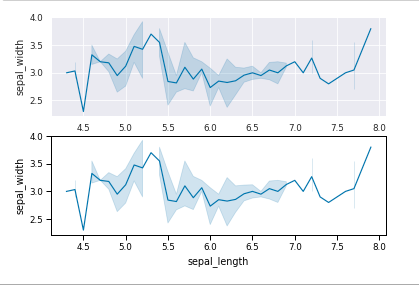Refer to the below article for detailed information about styling Seaborn Plot.

## Color Palette

Colormaps are used to visualize plots effectively and easily. One might use different sorts of colormaps for different kinds of plots. color_palette() method is used to give colors to the plot. Another function palplot() is used to deal with the color palettes and plots the color palette as a horizontal array.

Example:

## Python3

 `# importing packages ``import` `seaborn as sns ``import` `matplotlib.pyplot as plt`` ` `# current colot palette``palette ``=` `sns.color_palette()`` ` `# plots the color palette as a``# horizontal array``sns.palplot(palette)`` ` `plt.show()`

Output:### Diverging Color Palette

This type of color palette uses two different colors where each color depicts different points ranging from a common point in either direction. Consider a range of -10 to 10 so the value from -10 to 0 takes one color and values from 0 to 10 take another.

Example:

## Python3

 `# importing packages ``import` `seaborn as sns ``import` `matplotlib.pyplot as plt`` ` `# current colot palette``palette ``=` `sns.color_palette(``'PiYG'``, ``11``)`` ` `# diverging color palette``sns.palplot(palette)`` ` `plt.show()`

Output: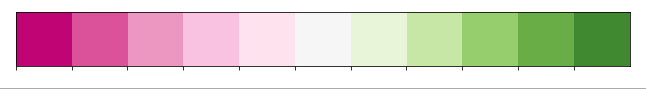In the above example, we have used an in-built diverging color palette which shows 11 different points of color. The color on the left shows pink color and color on the right shows green color.

### Sequential Color Palette

A sequential palette is used where the distribution ranges from a lower value to a higher value. To do this add the character ‘s’ to the color passed in the color palette.

Example:

## Python3

 `# importing packages ``import` `seaborn as sns ``import` `matplotlib.pyplot as plt`` ` `# current colot palette``palette ``=` `sns.color_palette(``'Greens'``, ``11``)`` ` `# sequential color palette``sns.palplot(palette)`` ` `plt.show()`

Output:### Setting the default Color Palette

set_palette() method is used to set the default color palette for all the plots. The arguments for both color_palette() and set_palette() is same. set_palette() changes the default matplotlib parameters.

Example:

## Python3

 `# importing packages ``import` `seaborn as sns ``import` `matplotlib.pyplot as plt`` ` `# loading dataset ``data ``=` `sns.load_dataset(``"iris"``) `` ` `def` `plot():``    ``sns.lineplot(x``=``"sepal_length"``, y``=``"sepal_width"``, data``=``data)`` ` `# setting the default color palette``sns.set_palette(``'vlag'``)``plt.subplot(``211``)`` ` `# plotting with the color palette``# as vlag``plot()`` ` `# setting another default color palette``sns.set_palette(``'Accent'``)``plt.subplot(``212``)``plot()`` ` `plt.show()`

Output: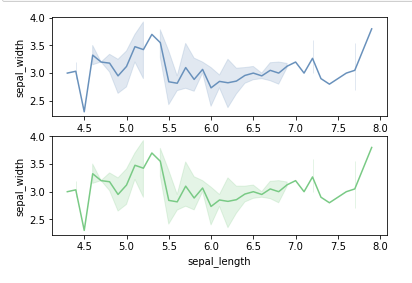Refer to the below article to get detailed information about the color palette.

## Multiple plots with Seaborn

You might have seen multiple plots in the above examples and some of you might have got confused. Don’t worry we will cover multiple plots in this section. Multiple plots in Seaborn can also be created using the Matplotlib as well as Seaborn also provides some functions for the same.

### Using Matplotlib

Matplotlib provides various functions for plotting subplots. Some of them are add_axes(), subplot(), and subplot2grid(). Let’s see an example of each function for better understanding.

Example 1: Using add_axes() method

## Python3

 `# importing packages``import` `seaborn as sns``import` `matplotlib.pyplot as plt`` ` ` ` `# loading dataset``data ``=` `sns.load_dataset(``"iris"``)`` ` `def` `graph():``    ``sns.lineplot(x``=``"sepal_length"``, y``=``"sepal_width"``, data``=``data)`` ` `# Creating a new figure with width = 5 inches``# and height = 4 inches``fig ``=` `plt.figure(figsize ``=``(``5``, ``4``)) `` ` `# Creating first axes for the figure``ax1 ``=` `fig.add_axes([``0.1``, ``0.1``, ``0.8``, ``0.8``]) `` ` `# plotting the graph``graph()`` ` `# Creating second axes for the figure``ax2 ``=` `fig.add_axes([``0.5``, ``0.5``, ``0.3``, ``0.3``])`` ` `# plotting the graph``graph()`` ` `plt.show()`

Output: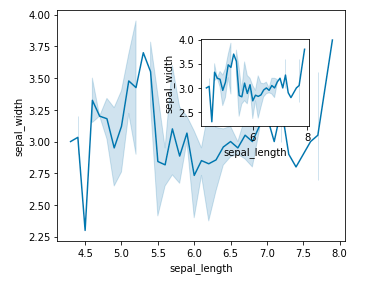Example 2: Using subplot() method

## Python3

 `# importing packages ``import` `seaborn as sns ``import` `matplotlib.pyplot as plt`` ` `# loading dataset ``data ``=` `sns.load_dataset(``"iris"``) `` ` `def` `graph():``    ``sns.lineplot(x``=``"sepal_length"``, y``=``"sepal_width"``, data``=``data)`` ` `# Adding the subplot at the specified``# grid position``plt.subplot(``121``)``graph()`` ` `# Adding the subplot at the specified``# grid position``plt.subplot(``122``)``graph()`` ` `plt.show()`

Output: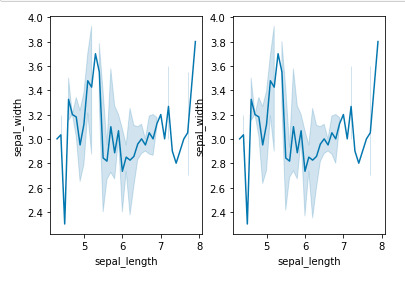Example 3: Using subplot2grid() method

## Python3

 `# importing packages``import` `seaborn as sns``import` `matplotlib.pyplot as plt`` ` `# loading dataset``data ``=` `sns.load_dataset(``"iris"``)`` ` `def` `graph():``    ``sns.lineplot(x``=``"sepal_length"``, y``=``"sepal_width"``, data``=``data)`` ` `# adding the subplots``axes1 ``=` `plt.subplot2grid (``  ``(``7``, ``1``), (``0``, ``0``), rowspan ``=` `2``,  colspan ``=` `1``) ``graph()`` ` `axes2 ``=` `plt.subplot2grid (``  ``(``7``, ``1``), (``2``, ``0``), rowspan ``=` `2``, colspan ``=` `1``) ``graph()``   ` `axes3 ``=` `plt.subplot2grid (``  ``(``7``, ``1``), (``4``, ``0``), rowspan ``=` `2``, colspan ``=` `1``)``graph()`

Output: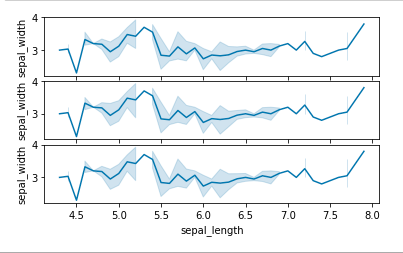### Using Seaborn

Seaborn also provides some functions for plotting multiple plots. Let’s see them in detail

Method 1: Using FacetGrid() method

• FacetGrid class helps in visualizing distribution of one variable as well as the relationship between multiple variables separately within subsets of your dataset using multiple panels.
• A FacetGrid can be drawn with up to three dimensions ? row, col, and hue. The first two have obvious correspondence with the resulting array of axes; think of the hue variable as a third dimension along a depth axis, where different levels are plotted with different colors.
• FacetGrid object takes a dataframe as input and the names of the variables that will form the row, column, or hue dimensions of the grid. The variables should be categorical and the data at each level of the variable will be used for a facet along that axis.

Syntax:

seaborn.FacetGrid( data, \*\*kwargs)

Example:

## Python3

 `# importing packages``import` `seaborn as sns``import` `matplotlib.pyplot as plt`` ` `# loading dataset``data ``=` `sns.load_dataset(``"iris"``)`` ` `plot ``=` `sns.FacetGrid(data, col``=``"species"``)``plot.``map``(plt.plot, ``"sepal_width"``)`` ` `plt.show()`

Output: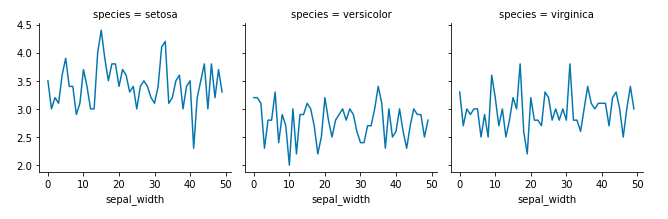Method 2: Using PairGrid() method

• Subplot grid for plotting pairwise relationships in a dataset.
• This class maps each variable in a dataset onto a column and row in a grid of multiple axes. Different axes-level plotting functions can be used to draw bivariate plots in the upper and lower triangles, and the marginal distribution of each variable can be shown on the diagonal.
• It can also represent an additional level of conventionalization with the hue parameter, which plots different subsets of data in different colors. This uses color to resolve elements on a third dimension, but only draws subsets on top of each other and will not tailor the hue parameter for the specific visualization the way that axes-level functions that accept hue will.

Syntax:

seaborn.PairGrid( data, \*\*kwargs)

Example:

## Python3

 `# importing packages``import` `seaborn as sns``import` `matplotlib.pyplot as plt`` ` `# loading dataset``data ``=` `sns.load_dataset(``"flights"``)`` ` `plot ``=` `sns.PairGrid(data)``plot.``map``(plt.plot)`` ` `plt.show()`

Output: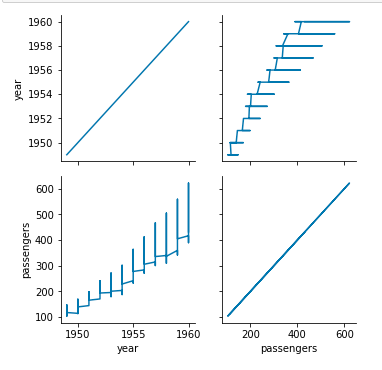Refer to the below articles to get detailed information about the multiple plots

## Relational Plots

Relational plots are used for visualizing the statistical relationship between the data points. Visualization is necessary because it allows the human to see trends and patterns in the data. The process of understanding how the variables in the dataset relate each other and their relationships are termed as Statistical analysis. Refer to the below articles for detailed information.

There are different types of Relational Plots. We will discuss each of them in detail –

### Relplot()

This function provides us the access to some other different axes-level functions which shows the relationships between two variables with semantic mappings of subsets. It is plotted using the relplot() method.

Syntax:

seaborn.relplot(x=None, y=None, data=None, **kwargs)

Example:

## Python3

 `# importing packages``import` `seaborn as sns``import` `matplotlib.pyplot as plt`` ` `# loading dataset``data ``=` `sns.load_dataset(``"iris"``)`` ` `# crrating the relplot``sns.relplot(x``=``'sepal_width'``, y``=``'species'``, data``=``data)`` ` `plt.show()`

Output: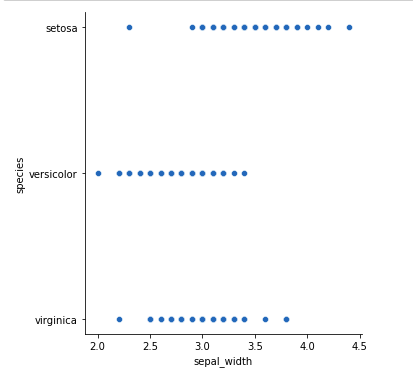### Scatter Plot

The scatter plot is a mainstay of statistical visualization. It depicts the joint distribution of two variables using a cloud of points, where each point represents an observation in the dataset. This depiction allows the eye to infer a substantial amount of information about whether there is any meaningful relationship between them. It is plotted using the scatterplot() method.

Syntax:

seaborn.scatterplot(x=None, y=None, data=None, **kwargs)

Example:

## Python3

 `# importing packages``import` `seaborn as sns``import` `matplotlib.pyplot as plt`` ` `# loading dataset``data ``=` `sns.load_dataset(``"iris"``)`` ` `sns.scatterplot(x``=``'sepal_length'``, y``=``'sepal_width'``, data``=``data)``plt.show()`

Output: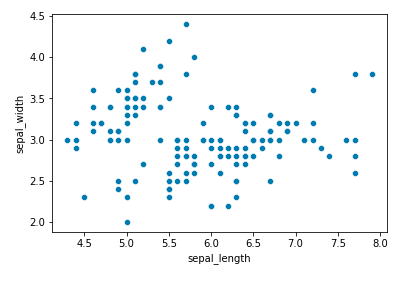Refer to the below articles to get detailed information about Scatter plot.

### Line Plot

For certain datasets, you may want to consider changes as a function of time in one variable, or as a similarly continuous variable. In this case, drawing a line-plot is a better option. It is plotted using the lineplot() method.

Syntax:

seaborn.lineplot(x=None, y=None, data=None, **kwargs)

Example:

## Python3

 `# importing packages``import` `seaborn as sns``import` `matplotlib.pyplot as plt`` ` `# loading dataset``data ``=` `sns.load_dataset(``"iris"``)`` ` `sns.lineplot(x``=``'sepal_length'``, y``=``'species'``, data``=``data)``plt.show()`

Output: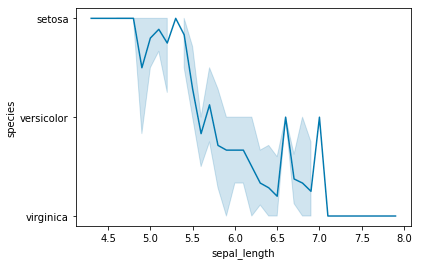Refer to the below articles to get detailed information about line plot.

## Categorical Plots

Categorical Plots are used where we have to visualize relationship between two numerical values. A more specialized approach can be used if one of the main variable is categorical which means such variables that take on a fixed and limited number of possible values.

Refer to the below articles to get detailed information.

There are various types of categorical plots let’s discuss each one them in detail.

### Bar Plot

A barplot is basically used to aggregate the categorical data according to some methods and by default its the mean. It can also be understood as a visualization of the group by action. To use this plot we choose a categorical column for the x axis and a numerical column for the y axis and we see that it creates a plot taking a mean per categorical column. It can be created using the barplot() method.

Syntax:

barplot([x, y, hue, data, order, hue_order, …])

Example:

## Python3

 `# importing packages``import` `seaborn as sns``import` `matplotlib.pyplot as plt`` ` `# loading dataset``data ``=` `sns.load_dataset(``"iris"``)`` ` `sns.barplot(x``=``'species'``, y``=``'sepal_length'``, data``=``data)``plt.show()`

Output: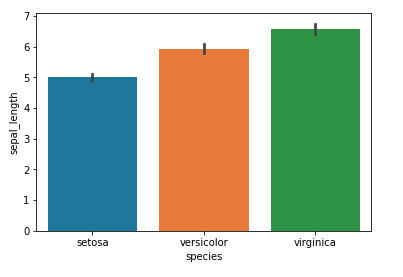Refer to the below article to get detailed information about the topic.

### Count Plot

A countplot basically counts the categories and returns a count of their occurrences. It is one of the most simple plots provided by the seaborn library. It can be created using the countplot() method.

Syntax:

countplot([x, y, hue, data, order, …])

Example:

## Python3

 `# importing packages``import` `seaborn as sns``import` `matplotlib.pyplot as plt`` ` `# loading dataset``data ``=` `sns.load_dataset(``"iris"``)`` ` `sns.countplot(x``=``'species'``, data``=``data)``plt.show()`

Output: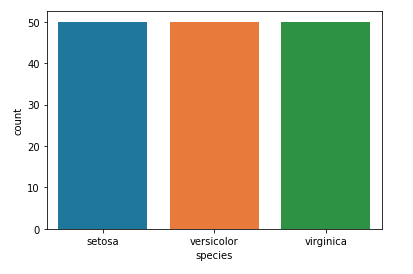Refer to the below articles t get detailed informaation about the count plot.

### Box Plot

A boxplot is sometimes known as the box and whisker plot.It shows the distribution of the quantitative data that represents the comparisons between variables. boxplot shows the quartiles of the dataset while the whiskers extend to show the rest of the distribution i.e. the dots indicating the presence of outliers. It is created using the boxplot() method.

Syntax:

boxplot([x, y, hue, data, order, hue_order, …])

Example:

## Python3

 `# importing packages``import` `seaborn as sns``import` `matplotlib.pyplot as plt`` ` `# loading dataset``data ``=` `sns.load_dataset(``"iris"``)`` ` `sns.boxplot(x``=``'species'``, y``=``'sepal_width'``, data``=``data)``plt.show()`

Output: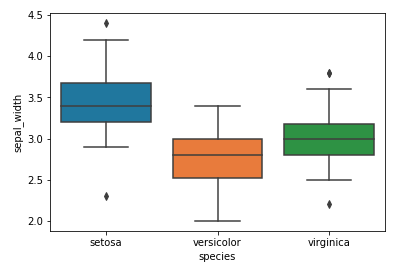Refer to the below articles to get detailed information about box plot.

### Violinplot

It is similar to the boxplot except that it provides a higher, more advanced visualization and uses the kernel density estimation to give a better description about the data distribution. It is created using the violinplot() method.

Syntax:

violinplot([x, y, hue, data, order, …]

Example:

## Python3

 `# importing packages``import` `seaborn as sns``import` `matplotlib.pyplot as plt`` ` `# loading dataset``data ``=` `sns.load_dataset(``"iris"``)`` ` `sns.violinplot(x``=``'species'``, y``=``'sepal_width'``, data``=``data)``plt.show()`

Output: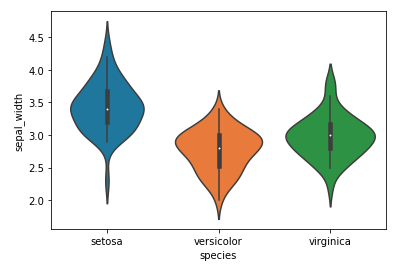Refer to the below articles to get detailed information about violin plot.

### Stripplot

It basically creates a scatter plot based on the category. It is created using the stripplot() method.

Syntax:

stripplot([x, y, hue, data, order, …])

Example:

## Python3

 `# importing packages``import` `seaborn as sns``import` `matplotlib.pyplot as plt`` ` `# loading dataset``data ``=` `sns.load_dataset(``"iris"``)`` ` `sns.stripplot(x``=``'species'``, y``=``'sepal_width'``, data``=``data)``plt.show()`

Output: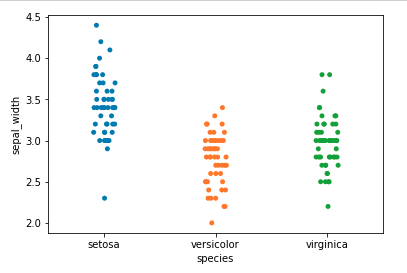Refer to the below articles to detailed information about strip plot.

### Swarmplot

Swarmplot is very similar to the stripplot except the fact that the points are adjusted so that they do not overlap.Some people also like combining the idea of a violin plot and a stripplot to form this plot. One drawback to using swarmplot is that sometimes they dont scale well to really large numbers and takes a lot of computation to arrange them. So in case we want to visualize a swarmplot properly we can plot it on top of a violinplot. It is plotted using the swarmplot() method.

Syntax:

swarmplot([x, y, hue, data, order, …])

Example:

## Python3

 `# importing packages``import` `seaborn as sns``import` `matplotlib.pyplot as plt`` ` `# loading dataset``data ``=` `sns.load_dataset(``"iris"``)`` ` `sns.swarmplot(x``=``'species'``, y``=``'sepal_width'``, data``=``data)``plt.show()`

Output: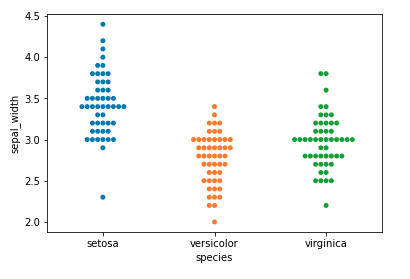Refer to the below articles to get deyailed information about swarmplot.

### Factorplot

Factorplot is the most general of all these plots and provides a parameter called kind to choose the kind of plot we want thus saving us from the trouble of writing these plots separately. The kind parameter can be bar, violin, swarm etc. It is plotted using the factorplot() method.

Syntax:

sns.factorplot([x, y, hue, data, row, col, …])

Example:

## Python3

 `# importing packages``import` `seaborn as sns``import` `matplotlib.pyplot as plt`` ` `# loading dataset``data ``=` `sns.load_dataset(``"iris"``)`` ` `sns.factorplot(x``=``'species'``, y``=``'sepal_width'``, data``=``data)``plt.show()`Refer to the below articles to get dtailed information about the factor plot.

## Distribution Plots

Distribution Plots are used for examining univariate and bivariate distributions meaning such distributions that involve one variable or two discrete variables.

Refer to the below article to get detailed informaon about the distribution plots.

There are various types of distribution plots let’s discuss each one them in detail.

### Histogram

A histogram is basically used to represent data provided in a form of some groups.It is accurate method for the graphical representation of numerical data distribution. It can be plotted using the histplot() function.

Syntax:

histplot(data=None, *, x=None, y=None, hue=None,  **kwargs)

Example:

## Python3

 `# importing packages``import` `seaborn as sns``import` `matplotlib.pyplot as plt`` ` `# loading dataset``data ``=` `sns.load_dataset(``"iris"``)`` ` `sns.histplot(x``=``'species'``, y``=``'sepal_width'``, data``=``data)``plt.show()`

Output: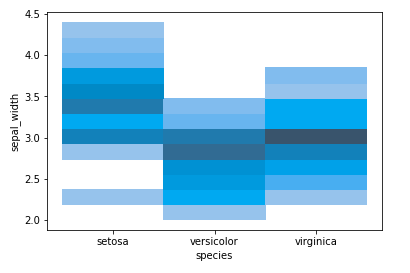Refer to the below articles to get detailed information about histplot.

### Distplot

Distplot is used basically for univariant set of observations and visualizes it through a histogram i.e. only one observation and hence we choose one particular column of the dataset. It is potted using the distplot() method.

Syntax:

distplot(a[, bins, hist, kde, rug, fit, …])

Example:

## Python3

 `# importing packages``import` `seaborn as sns``import` `matplotlib.pyplot as plt`` ` `# loading dataset``data ``=` `sns.load_dataset(``"iris"``)`` ` `sns.distplot(data[``'sepal_width'``])``plt.show()`

Output: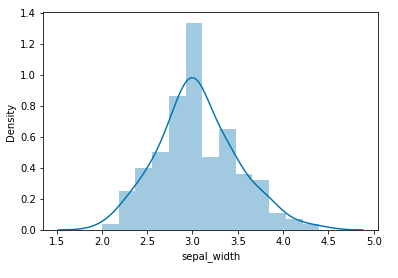### Jointplot

Jointplot is used to draw a plot of two variables with bivariate and univariate graphs. It basically combines two different plots. It is plotted using the jointplot() method.

Syntax:

jointplot(x, y[, data, kind, stat_func, …])

Example:

## Python3

 `# importing packages``import` `seaborn as sns``import` `matplotlib.pyplot as plt`` ` `# loading dataset``data ``=` `sns.load_dataset(``"iris"``)`` ` `sns.jointplot(x``=``'species'``, y``=``'sepal_width'``, data``=``data)``plt.show()`

Output: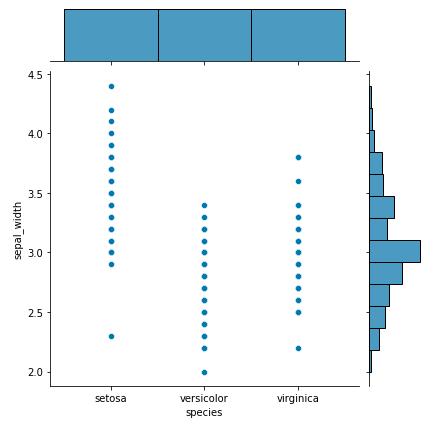Refer to the below articles to get detailed information about the topic.

### Pairplot

Pairplot represents pairwise relation across the entire dataframe and supports an additional argument called hue for categorical separation. What it does basically is create a jointplot between every possible numerical column and takes a while if the dataframe is really huge. It is plotted using the pairplot() method.

Syntax:

pairplot(data[, hue, hue_order, palette, …])

Example:

## Python3

 `# importing packages``import` `seaborn as sns``import` `matplotlib.pyplot as plt`` ` `# loading dataset``data ``=` `sns.load_dataset(``"iris"``)`` ` `sns.pairplot(data``=``data, hue``=``'species'``)``plt.show()`

Output: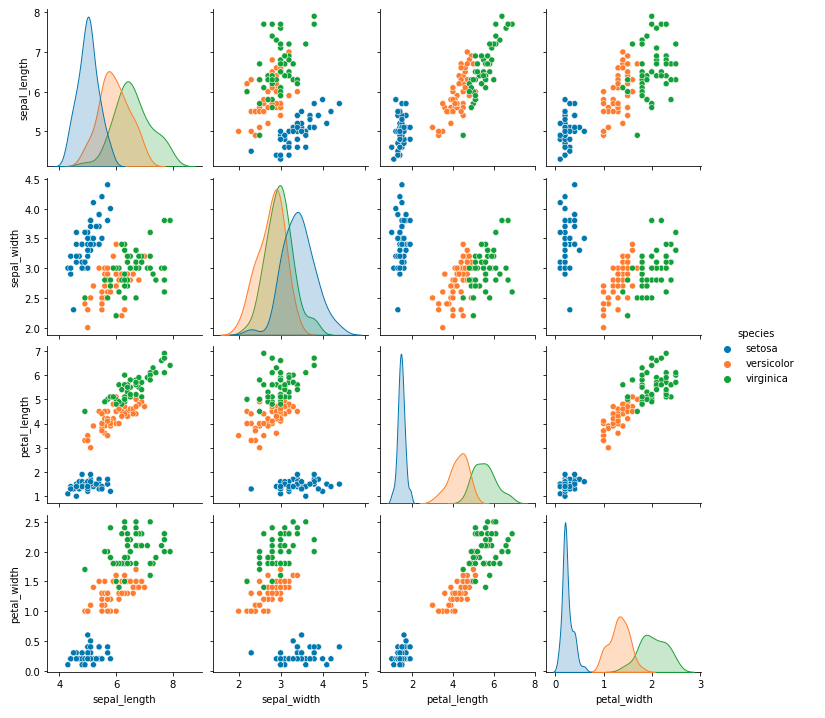Refer to the below articles to get deyailed information about the pairplot.

### Rugplot

Rugplot plots datapoints in an array as sticks on an axis.Just like a distplot it takes a single column. Instead of drawing a histogram it creates dashes all across the plot. If you compare it with the joinplot you can see that what a jointplot does is that it counts the dashes and shows it as bins. It is plotted using the rugplot() method.

Syntax:

rugplot(a[, height, axis, ax])

Example:

## Python3

 `# importing packages``import` `seaborn as sns``import` `matplotlib.pyplot as plt`` ` `# loading dataset``data ``=` `sns.load_dataset(``"iris"``)`` ` `sns.rugplot(data``=``data)``plt.show()`

Output: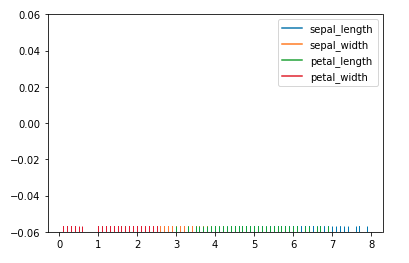### KDE Plot

KDE Plot described as Kernel Density Estimate is used for visualizing the Probability Density of a continuous variable. It depicts the probability density at different values in a continuous variable. We can also plot a single graph for multiple samples which helps in more efficient data visualization.

Syntax:

seaborn.kdeplot(x=None, *, y=None, vertical=False, palette=None, **kwargs)

Example:

## Python3

 `# importing packages``import` `seaborn as sns``import` `matplotlib.pyplot as plt`` ` `# loading dataset``data ``=` `sns.load_dataset(``"iris"``)`` ` `sns.kdeplot(x``=``'sepal_length'``, y``=``'sepal_width'``, data``=``data)``plt.show()`

Output: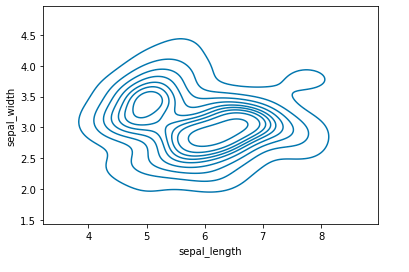Refer to the below articles to getdetailed information about the topic.

## Regression Plots

The regression plots are primarily intended to add a visual guide that helps to emphasize patterns in a dataset during exploratory data analyses. Regression plots as the name suggests creates a regression line between two parameters and helps to visualize their linear relationships.

Refer to the below article to get detailed information about the regression plots.

there are two main functions that are used to draw linear regression models. These functions are lmplot(), and regplot(), are closely reled to each other. They even share their core functionality.

### lmplot

lmplot() method can be understood as a function that basically creates a linear model plot. It creates a scatter plot with a linear fit on top of it.

Syntax:

seaborn.lmplot(x, y, data, hue=None, col=None, row=None, **kwargs)

Example:

## Python3

 `# importing packages``import` `seaborn as sns``import` `matplotlib.pyplot as plt`` ` `# loading dataset``data ``=` `sns.load_dataset(``"tips"``)`` ` `sns.lmplot(x``=``'total_bill'``, y``=``'tip'``, data``=``data)``plt.show()`

Output: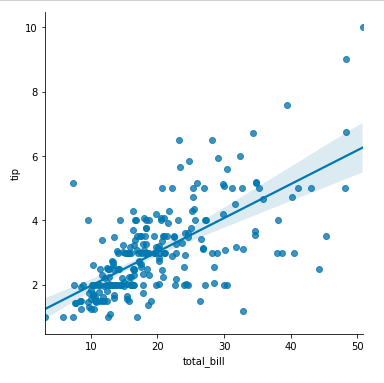Refer to the below articles to get detailed information about the lmplot.

### Regplot

regplot() method is also similar to lmplot which creates linear regression model.

Syntax:

seaborn.regplot( x,  y,  data=None, x_estimator=None, **kwargs)

Example:

## Python3

 `# importing packages``import` `seaborn as sns``import` `matplotlib.pyplot as plt`` ` `# loading dataset``data ``=` `sns.load_dataset(``"tips"``)`` ` `sns.regplot(x``=``'total_bill'``, y``=``'tip'``, data``=``data)``plt.show()`

Output: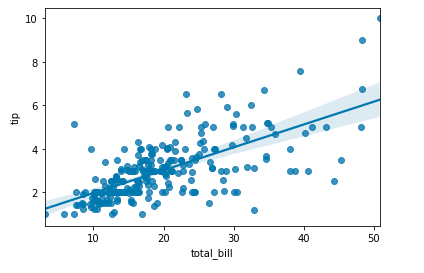Refer to the below articles to get detailed information about regplot.

Note: The difference between both the function is that regplot accepts the x, y variables in different format inlcuding NumPy arrays, Pandas objects, whereas, the lmplot only accepts the value as strings.

### Matrix Plots

A matrix plot means plotting matrix data where color coded diagrams shows rows data, column data and values. It can shown using the heatmap and clustermap.

Refer to the below articles to get detailed information about the matrix plots.

### Heatmap

Heatmap is defined as a graphical representation of data using colors to visualize the value of the matrix. In this, to represent more common values or higher activities brighter colors basically reddish colors are used and to represent less common or activity values, darker colors are preferred. it can be plotted using the heatmap() function.

Syntax:

seaborn.heatmap(data, *, vmin=None, vmax=None, cmap=None, center=None, annot_kws=None, linewidths=0, linecolor=’white’, cbar=True, **kwargs)

Example:

## Python3

 `# importing packages``import` `seaborn as sns``import` `matplotlib.pyplot as plt`` ` `# loading dataset``data ``=` `sns.load_dataset(``"tips"``)`` ` `# correlation between the different parameters ``tc ``=` `data.corr()`` ` `sns.heatmap(tc)``plt.show()`

Output: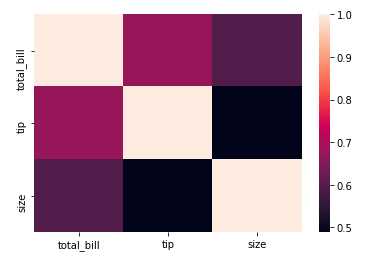Refer to the below articles to get detailed information about the heatmap.

### Clustermap

The clustermap() function of seaborn plots the hierarchically-clustered heatmap of the given matrix dataset. Clustering simply means grouping data based on relationship among the variables in the data.

Syntax:

clustermap(data, *, pivot_kws=None,  **kwargs)

Example:

## Python3

 `# importing packages``import` `seaborn as sns``import` `matplotlib.pyplot as plt`` ` `# loading dataset``data ``=` `sns.load_dataset(``"tips"``)`` ` `# correlation between the different parameters ``tc ``=` `data.corr()`` ` `sns.clustermap(tc)``plt.show()`

Output: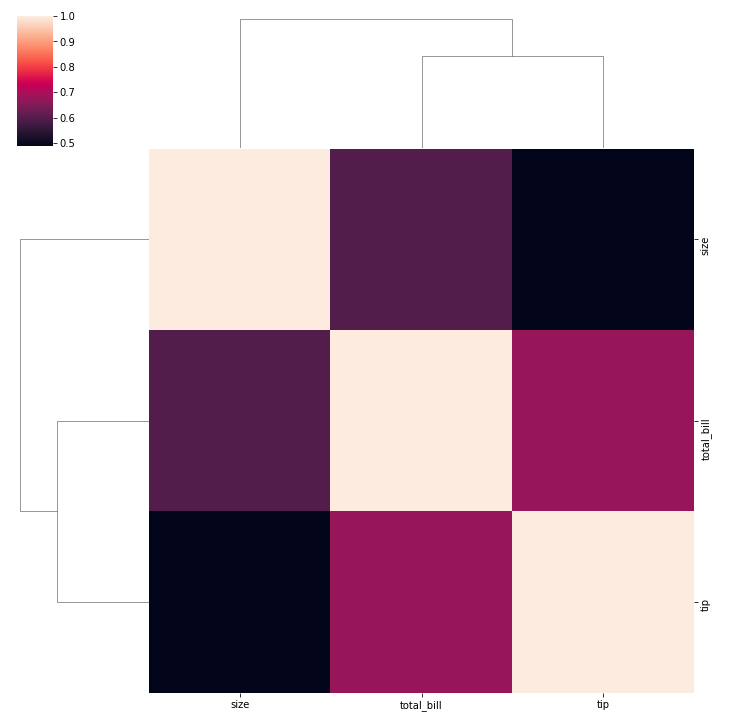Refer to the below articles to get detailed information about clustermap.

## More Topics on Seaborn

Attention geek! Strengthen your foundations with the Python Programming Foundation Course and learn the basics.

To begin with, your interview preparations Enhance your Data Structures concepts with the Python DS Course. And to begin with your Machine Learning Journey, join the Machine Learning – Basic Level Course

My Personal Notes arrow_drop_up